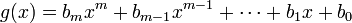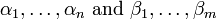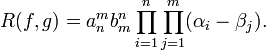# Resultant (algebra)

In algebra, the resultant of two polynomials is a quantity which determines whether or not they have a factor in common.

Given polynomials$f(x)= a_n x^n + a_{n-1} x^{n-1} + \cdots + a_1 x + a_0$

and$g(x)= b_m x^m + b_{m-1} x^{m-1} + \cdots + b_1 x + b_0$

with roots$\alpha_1,\ldots,\alpha_n \mbox{ and } \beta_1,\ldots,\beta_m$

respectively, the resultant R(f,g) is defined as$R(f,g) = a_n^m b_m^n \prod_{i=1}^n \prod_{j=1}^m (\alpha_i - \beta_j) .$

The resultant is thus zero if and only if f and g have a common root.

## Sylvester matrix

The Sylvester matrix attached to f and g is the square (m+n)×(m+n) matrix$\begin{pmatrix} a_n & a_{n-1} & \ldots & a_0 & 0 & \ldots & 0 \\ 0 & a_n & \ldots & a_1 & a_0 & \ldots & 0 \\ \vdots & \vdots & \ddots & \vdots & \vdots & \ddots & a_0 \\ b_n & b_{n-1} & \ldots & b_0 & 0 & \ldots & 0 \\ 0 & b_n & \ldots & b_1 & b_0 & \ldots & 0 \\ \vdots & \vdots & \ddots & \vdots & \vdots & \ddots & b_0 \end{pmatrix}$

in which the coefficients of f occupy m rows and those of g occupy n rows.

The determinant of the Sylvester matrix is the resultant of f and g.

The rows of the Sylvester matrix may be interpreted as the coefficients of the polynomials$x^{m-1} f(x), x^{m-2} f(x), \ldots, x f(x), f(x), x^{n-1} g(x), x^{n-2} g(x), \ldots, x g(x), g(x), \,$

and expanding the determinant we see that

R(f,g) = a(x)f(x) + b(x)g(x)

with a and b polynomials of degree at most m-1 and n-1 respectively, and R a constant (degree zero polynomial). If f and g have a polynomial common factor this must divide R and so R must be zero. Conversely if R is zero, then f/g = - b/a so f/g is not in lowest terms and f and g have a common factor.